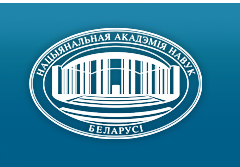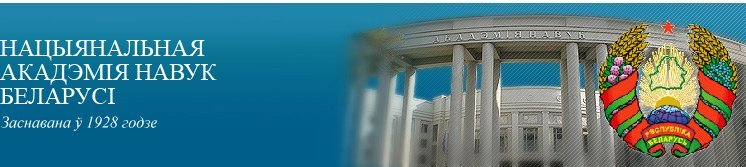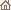Галоўная Навіны Аб акадэміі Дзейнасць акадэміі Члены акадэміі Арганізацыі Выданні акадэміі Мерапрыемствы Кантакты Электронныя звароты Даведачная інфармацыя Карта сайта

Весці Нац. акадэміі навук Беларусі. Сер. фіз.-матэм. навук, 2000, No.3/ Выданні акадэміі / Навуковыя часопісы

Весці Нац. акадэміі навук Беларусі. Сер. фіз.-матэм. навук, 2000, No.3Весці Нацыянальнай акадэміі навук Беларусі СЕРЫЯ ФІЗІКА-МАТЭМАТЫЧНЫХ НАВУКВыдавец: Інстытут матэматыкі НАН Беларусі, Мінск, Рэспубліка Беларусь

Нумар 3, 2000ЗМЕСТ

МАТЭМАТЫКА

Tsurko V. A.
Parabolic equations with discontinuous solutions. Difference schemes, approximation, convergence. pp. 5--10

Summary: Advancing difference schemes for both the linear and non-linear problems are constructed. Approximation estimates are obtained.

Pekarskii A. A.
Rational and piecewise polynomial approximations in the spaces Lp and Hp. pp. 11--16

Summary: Two-sided relations between best rational and best piecewise polynomial approximation in Lebesgue spaces Lp on the circle and Hardy Spaces Яр in the disc is given. These relations for the interval was obtained by V.A. Popov, P.P. Petrushev, H. Stahl and author.

Minchenko L. I., Sirotko S. I.
To necessary optimality conditions for discrete inclusions with delay. pp. 17--22

Summary: In this paper necessary optimality conditions are obtained for discrete inclusions-with delay which are based on adjoint multifunctions. This conditions extend the discrete maximum principle to the control problems for discrete inclusions with delay.

Kopeykina T. B.
On the question about complete controllability for linear stationary singularly perturbed systems with time delay. pp. 23--29

Summary: The problem of complete controllability for linear stationary singularly perturbed systems with time delay (LSSPSD) is considered. The D.K.Faddeev's method for simultaneous calculation of coefficients of characteristic polynomial and adjoint matrix is generalized for the matrix pencil C3 + mC4, where m is some parameter. Then this method is applied to get the reduced system for LSSPSD, Necessary and sufficient conditions of complete controllability for the reduced system and for the boundary layer system are proved.

Trubnikov Yu. V.
Optimum iterative processes of the second order. pp. 30--34

Summary: The iterative processes of a solution of the linear equations in Banach spaces based on construction of polynomials with minimum norm, determined on rectangle of a complex plane containing a spectrum of an operator are offered.

Beresnevich V. V.
On proof of an analog of the Khintchine theorem for curves. pp. 35--40

Summary: The paper contains a method of proving metrical Khintchine type theorems for curves and its applications.

Levina T. I., Matsevich D. A., Sinkevich D. V.
About two first moments of mistake one statistical model for estimation of transitional probabilities of a homogeneous Markov chain. pp. 41--46

Summary: Formulas of mathematical expectation and dispersion of mistake one statistical model for calculation of the estimation of transitional probabilities of a homogeneous Markov chain for the aggregated information are given.

Tishchenko K. I.
On some special cases of Wirsing's Conjecture. pp. 47--52

Summary: The following theorem is proved. New formulas for the estimation of the absolute value of nonzero integer polynomials at a given point are obtained as well.

Malafei D. A.
Difference schemes of high order of approximation on nonuniform grids for parabolic equation in curvilinear coordinates. pp. 53--57

Summary: Monotone difference schemes of the second oder of approximation with respect to space on an arbitrary irregular grid for one-dimensional parabolic equation in cylindrical and spherical coordinates are constructed and investigated. Stability and convergence in the norm of С are proved.

New construction of generalized functions and its application to the Hopf equation. pp. 58--62

Summary: The definition and construction of the algebra of generalized functions as classes of equivalent smooth functions is introduced. Functionals with values in nonarchimedean field of Laurent series is considered. Introduced objects applied to the definition of generalized solution of the equations ut + div f(u) = 0. Calculation method for the profile of infinitely narrow soliton and shock wave in case of the Hopf equation is proposed.

Prokhorovich T. N.
The differential matrices of the two-dimensional Fuch system with three singular points on the Riemann sphere. pp. 63--65

Summary: The differential matrices for linear system of the second order with Fuch features on the Riemann sphere are obtained in final solution. It is a special case of the monodromy group.

ФІЗІКА

Korshunov F. P., Lastovsky S. B., Bogatyrev Yu. V., Shesholko V. K.
Influence of electron irradiation on avalanche breakdown voltage of silicon p--n-junctions at 4.2--80 K. pp. 66--69

Summary: The influence of irradiation by electrons with energy 4 MeV on temperature dependencies of avalanche breakdown voltage of silicon p - n-junctions have been investigated in the range of temperatures 4.2--80 K. At initial and irradiated p - n-junctions the segments with non monotone temperature dependence of breakdown voltage are detected at 4.2--40 K, and also barrier capacity and conductivity at 22--65 K, stipulated by freezing of carriers on shallow impurity centers in quasi-neutral areas. Thus is established, that the introduction of radiation defects results in increase of a temperature coefficient of a breakdown voltage at 17--35 K, displacement of a maximum of conductivity and a segment of sharp decreasing of a capacity at 22--35 K to the field of higher temperatures. It is connected with influence of a degree of compensation n-area on low temperature conductivity of silicon.

Fedorovykh A. M.
Relativistic algebra of vectors and tensors and angular momentum conservation law. pp. 70--75

Summary: A set of binary operations over three-dimensional and four-dimensional vectors and tensors of rank two, which axe transformed by irreducible representations (1,0), (0,1), (1/2, 1/2), (2,0), (0,2), (3/2, 1/2), (1/2, 3/2) and (1,1) of the Lorentz group is constructed. It extends the operations of the ordinary three-dimensional vector algebra to the relativistic case. An algebraic formulation of the angular momentum conservation law is given in terms of the introduced operations.

Blinkouskii A. A., Gorbatsievich A. K.
Observational cosmological parameters in 5-dimensional Projective Unified Field Theory. pp. 76--80

Summary: The homogeneous and isotropic model of the universe is considered in the framework of 5-dimensional Projective Unified Field Theory in which the gravitation is described both with curvature of space-time and with some hypothetical scalar field. It is established that the presence of the scalar field leads with necessity to extension of number of the observational cosmological parameters in comparison with standard Friedmann model. It is shown that this circumstance can essentially facilitate a coordination of the observational data existing now.

Kisel' V. V.
Generally covariant generalization of the Cox equation for a particle with a spin 0 and possible display of its additional structure in gravitational interactions. pp. 81--85

Summary: Generally covariant Cox equation for a massive particle with spin 0 is considered. It is found that this equation can reduce to the generally covariant Duffin -- Kemmer equation with the same spin and mass which includes an additional, previously not considered term that describes interaction with external gravitational field. This term may be caused some additional structure of the particle which manifests itself in gravitational interactions.

Zhestkov S. V., Kuvshinov V. I.
Multi-dimentional variant of Hirota's method for sin-Gordon equation. pp. 86--90

Summary: It is found that Hirota's method can be used for multi-dimensional sin-Gordon equations. Single and double exponential solutions are constructed. Conditions are obtained at which the double exponential solution describes interaction of two single exponential solutions.

Rutkowskii F. K.
Errors of ray trajectory calculation in gradan. pp. 91--96

Summary: Two variants of differential equations for ray trajectory are solved by power series expansion to fourth order. Exactness is estimated by the value of error determined by halving the step and by the difference from zero of operator •which equals to zero for exact solution. For parametric variant in which three coordinates of ray point are determined as functions of ray arc length the desired exactness is reached much easier than for variant in which two coordinates are determined as functions of third coordinate.

Khilo P. A.
Light diffraction non-linear control of ultrasonic waves. pp. 97--99

Summary: The problem of nonlinear optical control by the process of ultrasonic wave light diffraction is solved. The control is made by means of nonlinear phase modulation effect of transmitted and diffracted waves.

Belevich N. N., Igumentsev A. N., Makovetskii G. I.
Peparation of CuInSe2 films by an evaporation from a single source and their properties. pp. 100--104

Summary: The chemical composition and optical properties of films CuInSe2 obtained by a method of thermal evaporation from a single source of mixture of the compound.

Tochitskii Т. A., Nemtsevich L. V.
The investigation of the formation of the perpendicular magnetic anisotropy in electrolytic Co-based films. pp. 105--109

Summary: The formation of the perpendicular magnetic anisotropy has been investigated during crystalline to amorphous transition in electrolytic Co-based films.

Korostik K. N., Polyakov A. V.
Mathematical model for development of optoelectronic dynamic memory devices with the given parameters. pp. 110--115

Summary: On the basis of the developed mathematical model the multiparametrical analysis of the characteristics of the optoelectronic dynamic memory device with periodic regeneration of an data stream is carried out. The influence of operation modes of contour elements on parameters of the system have been investigated.

ІНФАРМАТЫКА

Demidenko V. M.
The description of cone of Kalmanson's matrices in the space of minimum dimension. pp. 116--122

Summary: It is obtained the minimal description and enumerated in the explicit form the minimal proper surfaces of the cone of symmetrical Kalmanson's matrix, for which the strong solvable of traveling salesman problem are guaranteed.

Malenko O. G., Sadykhov R. Kh.
The method of self-organizing of training and classification in the recognition of handwriten. pp. 123--127

Summary: The algorithm of the classificator training which use the structural methods is proposed. The training can be realized on the base of self-organizing of the ideal description classes. This approach allows to choose the minimal set, covering the all space of classes.

Klimenok V. I.
On the steady-state condition for the retrial queuing systems with the batch Markov arrival process. pp. 128--132

Summary: The sufficient condition for stationary distribution existence in retrial BMAP|SM|1 queue is obtained.

КАРОТКІЯ ПАВЕДАМЛЕННІ

Yudin M. D.
About approximating of distributions of the sums of dependent random vectors when the coordinates of the sums are independent. pp. 133--135

Summary: The distribution of the sums of mn-dependent random vectors is approximated by the infinitely distribution if the coordinates sums independent.

АНАТАЦЫІ ДЭПАНІРАВАНЫХ АРТЫКУЛАЎ

Emelichev V. A., Pashkevich A. V.
On vector, discrete, major optimization problems solvable with help of the linear convolution of tests. pp. 136--136

Smolik Ch. K.
Stable method of the solving of the one-dimensional linear integral Fredholm equations of the first type for the exact input data. pp. 136--136.

ВУЧОНЫЯ БЕЛАРУСI

Андрэй Аляксандравіч Богуш (Да 75-годдзя з дня нараджэння). C. 137--138
Націсніце
тут, каб прачытаць артыкул/ Выданні акадэміі / Навуковыя часопісы / Да пачатку старонкі

Распрацавана і падтрымліваецца Мікалаем М. Касцюковічам. Апошняе абнаўленне: 8 снежня 2006 г.
Створана пры ўдзеле Ігната І. Корсака
Капірайт © 2000-2006 Нацыянальная акадэмія навук Беларусі
Капірайт © 2000 Інстытут матэматыкі НАН Беларусі